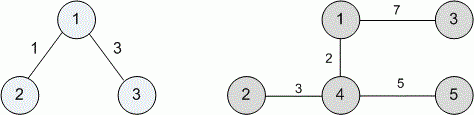Problems

# Sum of distances

Time limit 1 second
Memory limit 128 MiB

You are given a weighted tree with n vertices and n - 1 edges. The distance between the vertices u and v is the weight of the smallest edge on the path between u and v.

Find the sum of the distances between all pairs of tree vertices.

## Input data

The first line contains the single integer number n~(2 \le n \le 10^5) — the number of vertexes in graph. It is followed by n - 1 lines containing the description of the edges. Every line of description consists of three integer numbers: vertices connected by the edge (labeled from 1 to n) and edge’s weight.

## Output data

Print the sum of the distances between all pairs of tree vertices.## Examples

Input example #1
3
1 2 1
1 3 3

Output example #1
5

Input example #2
5
1 3 7
4 1 2
4 5 5
2 4 3

Output example #2
30

Author Michael Medvediev